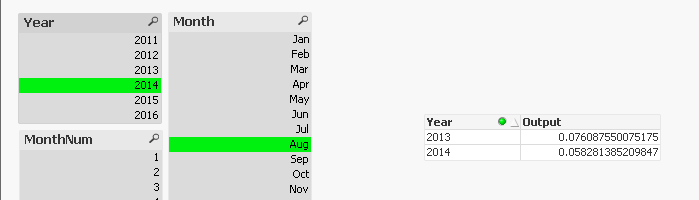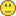# QlikView App Development

Discussion Board for collaboration related to QlikView App Development.

Contributor III

## How to calculate MAT when year as a dimension

Hi Guys,

I need to calculate MAT for the available data

Moving Average Turnover (MAT):

Dimensions: Year

Expression : (Current Year - Previous Year) / Previous Year

Current Year : latest 12 months i.e.,(Aug-15 to Jul-16)

Previous Year : previous latest 12 months i.e.,(Aug-14 to Jul-15)

Expression output value sholud be plotted in latest year i.e., 2016

Need to show all the Year

Example 1:

Dimension      Expression

2016           (Current Year (Aug-15 to Jul-16) - Previous Year (Aug-14 to Jul-15)) / Previous Year (Aug-14 to Jul-15))

2015           (Current Year (Aug-14 to Jul-15) - Previous Year (Aug-13 to Jul-14)) / Previous Year (Aug-13 to Jul-14))

sooo on for the remaining years

Month interval has to vary based on the selection

Exanple 2:

Selection: Year= 2016 Month= Apr

Dimension      Expression

2016           (Current Year (May-15 to Apr-16) - Previous Year (May-14 to Apr-15)) / Previous Year (May-14 to Apr-15))

2015           (Current Year (May-14 to Apr-15) - Previous Year (May-13 to Apr-14)) / Previous Year (May-13 to Apr-14))

sooo on for the remaining years

Selection: Year= 2015

Dimension      Expression

2015           (Current Year (Jan-15 to Dec-15) - Previous Year (Jan-14 to Dec-14)) / Previous Year (Jan-14 to Dec-14))

2014           (Current Year (Jan-14 to Dec-14) - Previous Year (Jan-13 to Dec-13)) / Previous Year (Jan-13 to Dec-13))

sooo on for the remaining years

Attached the same data

Tags (4)
1 Solution

Accepted SolutionsMVP

## Re: How to calculate MAT when year as a dimension

You want to see less then the years selected? May be try this:

FirstSortedValue({<MonthNum = {\$(=Max({<Year = {\$(=Max(Year))}>}MonthNum))}, Year = {"\$(='<=' & Max(Year))"}, Month>}Aggr(RangeSum(Above(Sum({<Year, Month>}Sales), 0, 12)), YearMonth), -Aggr(Only({<Year, Month>}YearMonth), YearMonth))

/

Above(FirstSortedValue({<MonthNum = {\$(=Max({<Year = {\$(=Max(Year))}>}MonthNum))}, Year, Month>}Aggr(RangeSum(Above(Sum({<Year, Month>}Sales), 0, 12)), YearMonth), -Aggr(Only({<Year, Month>}YearMonth), YearMonth)))-124 Replies
Contributor III

## Re: How to calculate MAT when year as a dimension

Sorry Guys

I attached a worng QVW

Pls have a look on the corrected attachment below

Regards

HemanthMVP

## Re: How to calculate MAT when year as a dimension

May be this?

FirstSortedValue({<MonthNum = {\$(=Max({<Year = {\$(=Max(Year))}>}MonthNum))}>}Aggr(RangeSum(Above(Sum(Sales), 0, 12)), YearMonth), -YearMonth)

/

Above(FirstSortedValue({<MonthNum = {\$(=Max({<Year = {\$(=Max(Year))}>}MonthNum))}>}Aggr(RangeSum(Above(Sum(Sales), 0, 12)), YearMonth), -YearMonth))-1Contributor III

## Re: How to calculate MAT when year as a dimension

Hi Sunny,

Exactly the same answer which i required.

But still one more required when i select the year the data is not displayed in the chart

please sort out this oneMVP

## Re: How to calculate MAT when year as a dimension

Ignore selection in Year field my friend. Have you not looked at set analysis guide yet? Highly recommend spending some time with it-> Set Analysis: syntaxes, examples

FirstSortedValue({<MonthNum = {\$(=Max({<Year = {\$(=Max(Year))}>}MonthNum))}, Year>}Aggr(RangeSum(Above(Sum({<Year>}Sales), 0, 12)), YearMonth), -YearMonth)

/

Above(FirstSortedValue({<MonthNum = {\$(=Max({<Year = {\$(=Max(Year))}>}MonthNum))}, Year>}Aggr(RangeSum(Above(Sum({<Year>}Sales), 0, 12)), YearMonth), -YearMonth))-1

Contributor III

## Re: How to calculate MAT when year as a dimension

Oops i forget it, Highly stressed in mind might forget small small things

But these forget things can be over come when there is a friend like you.Cheers....!MVP

## Re: How to calculate MAT when year as a dimension

You got it brotherContributor III

## Re: How to calculate MAT when year as a dimension

Hi Sunny,

If u dont mine can please explain the expression a bit clear

actually what happened is the expression is working very fine with my sample data

But when i implemented the same expression in my original data  it is not working

even i tired step by step too

firstsortedvalue function is not working  in the original data

can u pls help me

Regards

Hemanth

Contributor III

## Re: How to calculate MAT when year as a dimension

Hi Sunny,

Actually what is issue is

In the sample data , For one month there is only one value

ex:

YearMonth    Sale

Apr-16          201

May-16          301

But I my actual data, for one month there are so many values

Ex:

Yearmonth        Sale

Apr-16          201

Apr-16          202

Apr-16          203

Apr-16          204

May-16          301

May-16          302

May-16          303

May-16          304

May-16          305

i think because of this the firstsortedvalue function hasnt work

is there any other suggestion to overcome this issue

Regards

HemanthMVP

## Re: How to calculate MAT when year as a dimension

Would you be able to update the sample and show me the issue?